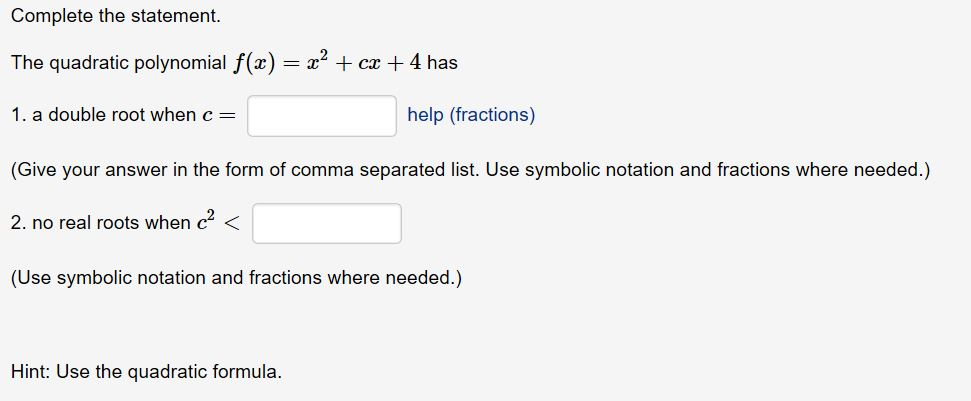# Complete the statementThe quadratic polynomial f(x) = x2 + cx + 4 has1. a double root when c(Give your answer in the form of comma separated list. Use symbolic notation and fractions where needed.)2, no real roots when c2 <(Use symbolic notation and fractions where needed.)help (fractions)Hint: Use the quadratic formula.

Question
4 views

Help.help_outlineImage TranscriptioncloseComplete the statement The quadratic polynomial f(x) = x2 + cx + 4 has 1. a double root when c (Give your answer in the form of comma separated list. Use symbolic notation and fractions where needed.) 2, no real roots when c2 < (Use symbolic notation and fractions where needed.) help (fractions) Hint: Use the quadratic formula. fullscreen
check_circle

Step 1

Given :

Step 2

As we know that

has roots of the form

Step 3

### Want to see the full answer?

See Solution

#### Want to see this answer and more?

Solutions are written by subject experts who are available 24/7. Questions are typically answered within 1 hour.*

See Solution
*Response times may vary by subject and question.
Tagged in

### Other